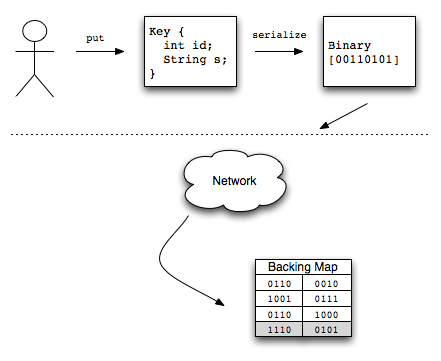# ~pperalta

Thoughts on software development and other stuff

## Coherence Key HOWTOOn occasion I am asked about best practices for creating classes to be used as keys in Coherence. This usually comes about due to unexpected behavior that can be explained by incorrect key implementations.

First and foremost, `equals` and `hashCode` need to be implemented correctly for any type used as a key. I won’t describe how to do this – instead I’ll defer to Josh Bloch who has written the definitive guide on this topic.

There is an additional requirement that needs to be addressed. All serializable (non transient) fields in the key class must be used in the `equals` implementation. To understand this requirement, let’s explore how Coherence works behind the scenes.

First, let’s try the following experiment:

```public class Key implements Serializable { public Key(int id, String zip) { m_id = id; m_zip = zip; }   //...   @Override public boolean equals(Object o) { // print stack trace new Throwable("equals debug").printStackTrace(); if (this == o) { return true; } if (o == null || getClass() != o.getClass()) { return false; }   Key key = (Key) o;   if (m_id != key.m_id) { return false; } if (m_zip != null ? !m_zip.equals(key.m_zip) : key.m_zip != null) { return false; } return true; }   @Override public int hashCode() { // print stack trace new Throwable("hashCode debug").printStackTrace(); int result = m_id; result = 31 * result + (m_zip != null ? m_zip.hashCode() : 0); return result; }   private int m_id; private String m_zip; }```

This key prints out stack traces in `equals` and `hashCode`. Now use this key with a HashMap:

```public static void testKey(Map m) { Key key = new Key(1, "12345");   m.put(key, "value"); m.get(key); }   //...   testKey(new HashMap());```

Output is as follows:

```java.lang.Throwable: hashCode debug at oracle.coherence.idedc.Key.hashCode(Key.java:60) at java.util.HashMap.put(HashMap.java:372) at oracle.coherence.idedc.KeyTest.testKey(KeyTest.java:46) at oracle.coherence.idedc.KeyTest.testKey(KeyTest.java:52) at oracle.coherence.idedc.KeyTest.main(KeyTest.java:18) java.lang.Throwable: hashCode debug at oracle.coherence.idedc.Key.hashCode(Key.java:60) at java.util.HashMap.get(HashMap.java:300) at oracle.coherence.idedc.KeyTest.testKey(KeyTest.java:47) at oracle.coherence.idedc.KeyTest.testKey(KeyTest.java:52) at oracle.coherence.idedc.KeyTest.main(KeyTest.java:18)```

Try it again with a partitioned cache this time:

`testKey(CacheFactory.getCache("dist-test"));`

Note the absence of stack traces this time. Does this mean Coherence is not using the key’s `equals` and `hashCode`? The short answer (for now) is yes. Here is the flow of events that occur when executing a put with a partitioned cache:

1. Invoke NamedCache.put
2. Key and value are serialized
3. Hash is executed on serialized key to determine which partition the key belongs to
4. Key and value are transferred to the storage node (likely over the network)
5. Cache entry is placed into backing map in binary formNote that objects are not deserialized before placement into the backing map – objects are stored in their serialized binary format. As a result, this means that two keys that are equal to each other in object form must be equal to each other in binary form so that the keys can be later be used to retrieve entries from the backing map. The most common way to violate this principle is to exclude non transient fields from `equals`. For example:

```public class BrokenKey implements Serializable { public BrokenKey(int id, String zip) { m_id = id; m_zip = zip; }   //...   @Override public boolean equals(Object o) { if (this == o) { return true; } if (o == null || getClass() != o.getClass()) { return false; }   BrokenKey brokenKey = (BrokenKey) o;   if (m_id != brokenKey.m_id) { return false; }   return true; }   @Override public int hashCode() { int result = m_id; result = 31 * result; return result; } }```

Note this key has two fields (id and zip) but it only uses id in the `equals`/`hashCode` implementation. I have the following method to test this key:

```public static void testBrokenKey(Map m) { BrokenKey keyPut = new BrokenKey(1, "11111"); BrokenKey keyGet = new BrokenKey(1, "22222");   m.get(keyPut); m.put(keyPut, "value");   System.out.println(m.get(keyPut)); System.out.println(m.get(keyGet)); }```

Output using HashMap:

```value value```

Output using partitioned cache:

```value null```

This makes sense, since `keyPut` and `keyGet` will serialize to different binaries. However, things get really interesting when combining partitioned cache with a near cache. Running the example using a near cache gives the following results:

```value value```

What happened? In this case, the first get resulted in a near cache miss, resulting in a read through to the backing partitioned cache. The second get resulted in a near cache hit because the object’s equals/hashCode was used (since near caches store data in object form.)

In addition to `equals`/`hashCode`, keep the following in mind:

• Keys should be immutable. Modifying a key while it is in a map generally isn’t a good idea, and it certainly won’t work in a distributed/partitioned cache.
• Key should be as small as possible. Many operations performed by Coherence assume that keys are very light weight (such as the key based listeners that are used for near cache invalidation.)
• Built in types (String, Integer, Long, etc) fit all of this criteria. If possible, consider using one of these existing classes.)

Written by Patrick Peralta

June 6th, 2010 at 10:28 pm